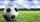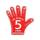Elections

In elections candidate 10 political parties. Calculate how many possible ways can the elections finish, if any two parties will not get the same number of votes.

Result

n =  3628800

Solution:Leave us a comment of example and its solution (i.e. if it is still somewhat unclear...):Be the first to comment!To solve this verbal math problem are needed these knowledge from mathematics:

See also our variations calculator. Would you like to compute count of combinations?

Next similar examples:

1. ChordsHow many 4-tones chords (chord = at the same time sounding different tones) is possible to play within 7 tones?
2. Olympics metalsIn how many ways can be win six athletes medal positions in the Olympics? Metal color matters.
3. MedalsIn how many ways can be divided gold, silver and bronze medal among 21 contestant?
4. ConfectioneryThe village markets have 5 kinds of sweets, one weighs 31 grams. How many different ways a customer can buy 1.519 kg sweets.
5. Football leagueIn the football league is 16 teams. How many different sequence of results may occur at the end of the competition?
6. PIN - codesHow many five-digit PIN - code can we create using the even numbers?
7. NumbersHow many different 7 digit natural numbers in which no digit is repeated, can be composed from digits 0,1,2,3,4,5,6?
8. Three workshopsThere are 2743 people working in three workshops. In the second workshop works 140 people more than in the first and in third works 4.2 times more than the second one. How many people work in each workshop?
9. Theorem proveWe want to prove the sentence: If the natural number n is divisible by six, then n is divisible by three. From what assumption we started?
10. VariationsDetermine the number of items when the count of variations of fourth class without repeating is 42 times larger than the count of variations of third class without repetition.
11. Reference angleFind the reference angle of each angle:
12. TrigonometryIs true equality? ?
13. SequenceWrite the first 6 members of these sequence: a1 = 5 a2 = 7 an+2 = an+1 +2 an
14. SequenceWrite the first 7 members of an arithmetic sequence: a1=-3, d=6.
15. Variations 4/2Determine the number of items when the count of variations of fourth class without repeating is 600 times larger than the count of variations of second class without repetition.
16. ValueFind the value of the expression: 6!·10^-3
17. Sequence 2Write the first 5 members of an arithmetic sequence a11=-14, d=-1